1596675060

# The statistics of Blackjack

Considered as the casino game with the lowest edge for the house, gamblers are supposed to have more chances at winning at Blackjack than at any other game. But what are the odds? And how do we find the optimal strategy to apply?

Let’s find out through a simple statistical analysis!

# Setting the scene

This article exploits a simulation tool for Blackjack built with **R **to deduce an optimal strategy and the associated probabilities.

All codes are available on Github.

## ArnaudBu/blackjack_simulation

Simulations of blackjack games. Contribute to ArnaudBu/blackjack_simulation development by creating an account on…

github.com

The rules used for the simulations are:

• Each player, including the dealer, starts with two cards. One of the dealer’s cards is hidden and will be revealed at the end of the round, when comes the dealer’s turn to play.
• The goal is to ask for cards to beat the dealer’s hand without exceeding 21, each card accounting for its nominal value (Kings, Queens, and Jacks are worth 10). Aces are worth 1 or 11, whichever value gives the best score without busting. If a hand has an ace whose value is 11, it is called soft, and the opposite is called a hard hand.
• If the player exceeds 21 (bust), no matter the dealer’s score, the dealer wins the bet. If the dealer busts and the player does not, the player wins. In case of similar scores under 21, the round results in a draw. In all other cases, the higher score wins the round.
• The dealer pays the bet 1 to 1, except for a natural Blackjack (Ace + card whose value is 10) that pays 3 to 2.
• At each move, the player can _hit _(ask for a card), _stand _(remain at its current position), or _double _(the bet is doubled but only one more card will be drawn).
• When the player has two identical cards, he can split, which means he can transform his pair into two separate hands that will be played independently.

The script `[run.R](https://github.com/ArnaudBu/blackjack_simulation/blob/master/run.R)` simulates Blackjack games (based on 10,000,000 moves with 8 decks and 3 people playing) to generate a database that will be analyzed with the data.table package.

The dataset produced follows the format below, with each line representing a move in a round (game_id) with the expected earning in case the player hits, stands, or doubles. The hard_if_hit value indicates if the hand is hard after the move and is necessary for the optimal strategy definition.

``````	score	score_dealer	hard	score_if_hit	score_fin_dealer	game_id	hit	stand	double	hard_if_hit
5	10	TRUE	11	19	4	-1	-1	-2	TRUE
11	10	TRUE	20	19	4	1	-1	2	TRUE
20	10	TRUE	30	19	4	-1	1	-2	TRUE
14	10	TRUE	24	19	5	-1	-1	-2	TRUE
14	9	TRUE	24	19	6	-1	-1	-2	TRUE
15	9	FALSE	19	19	7	0	-1	0	FALSE
19	9	FALSE	16	19	7	-1	0	-2	TRUE
16	9	TRUE	18	19	7	-1	-1	-2	TRUE
18	9	TRUE	19	19	7	0	-1	0	TRUE
19	9	TRUE	29	19	7	-1	0	-2	TRUE
view raw
blackjack_games_examples.csv hosted with ❤ by GitHub
``````

Table 1 — Blackjack analytics dataset

All analyses performed are available in the `[analysis.R](https://github.com/ArnaudBu/blackjack_simulation/blob/master/analysis.R)` script.

# Basic strategy

The most basic strategy one could think about is standing once the score reaches a certain threshold.

In terms of code, we just need to filter the dataset to retrieve one line by _game_id _that corresponds to the first score that exceeds the threshold, or the first _score_if_hit _that does so if the first condition is not met.

Applying this strategy to the dataset for each threshold from 2 to 21, we get the following results after aggregation.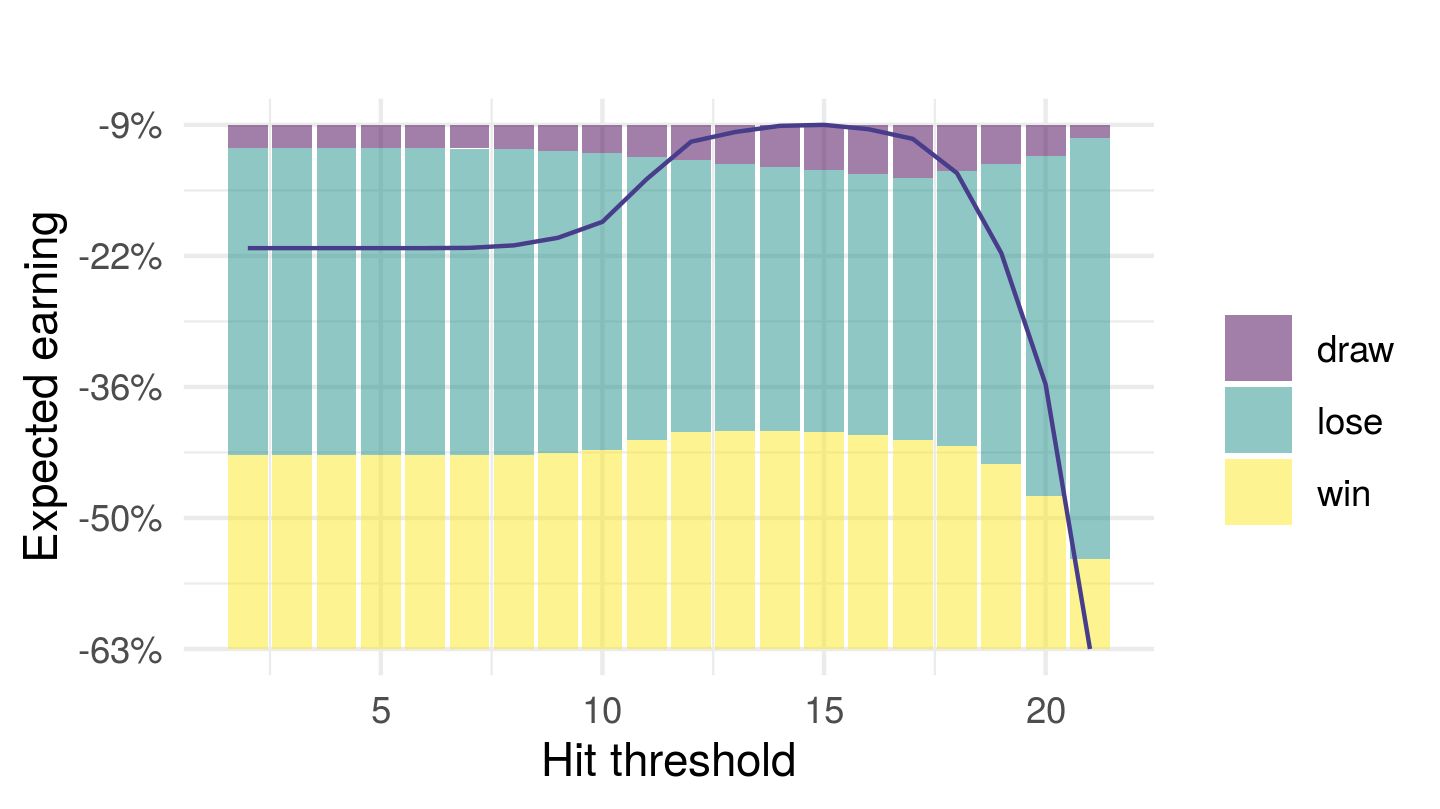Figure 1 — Hit threshold strategy returns

The graph above represents the expected earnings for each round (in percent of the gambled amount) with the distribution of outcomes.

The optimal strategy uses a threshold of 15, for an expected loss of 8.57 % of the gambled amount at each round. Those odds would obviously lead the game to end prematurely for most players.

It is, fortunately, possible to design a strategy capable of improving these odds by taking into account the dealer’s score and the soft aces in the player’s hand in the decision process.

# The optimal strategy

To find the optimal strategy, we first need a metric for optimization. We will use the expected earnings after a move.

Computing this metric for a given hand (score & if the hand is soft), requires knowing:

• the next possible hands when hitting, with the probability to transition to each one of them;
• the associated expected earnings for those hands, based on the moves given by the strategy we aim at defining.

We thus have a recursive problem to handle, since we first need to estimate the possible later moves of the round, dealer’s score being constant. This requires implementing a loop going** backward on scores. **Nevertheless, the possibility to have a hard or soft hand implies to also consider this when ordering the steps.

The following chart describes the transitions between hands.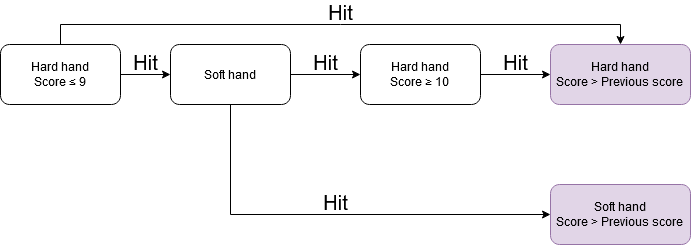Figure 2 — Possibilities when hitting

If the player has a hard hand with a score higher than 10, he can only get a hard hand with a higher score when hitting. A soft hand can transform into a soft hand with a higher score or a hard hand with a score higher than 10. Finally, a hard hand with a score lower than 9 can give a soft hand or a hard hand with a higher score. This means that we need to sequence our backward loop with three pivotal steps.

#data-analytics #r #simulation #blackjack #data-visualization #data analysis

## Buddha Community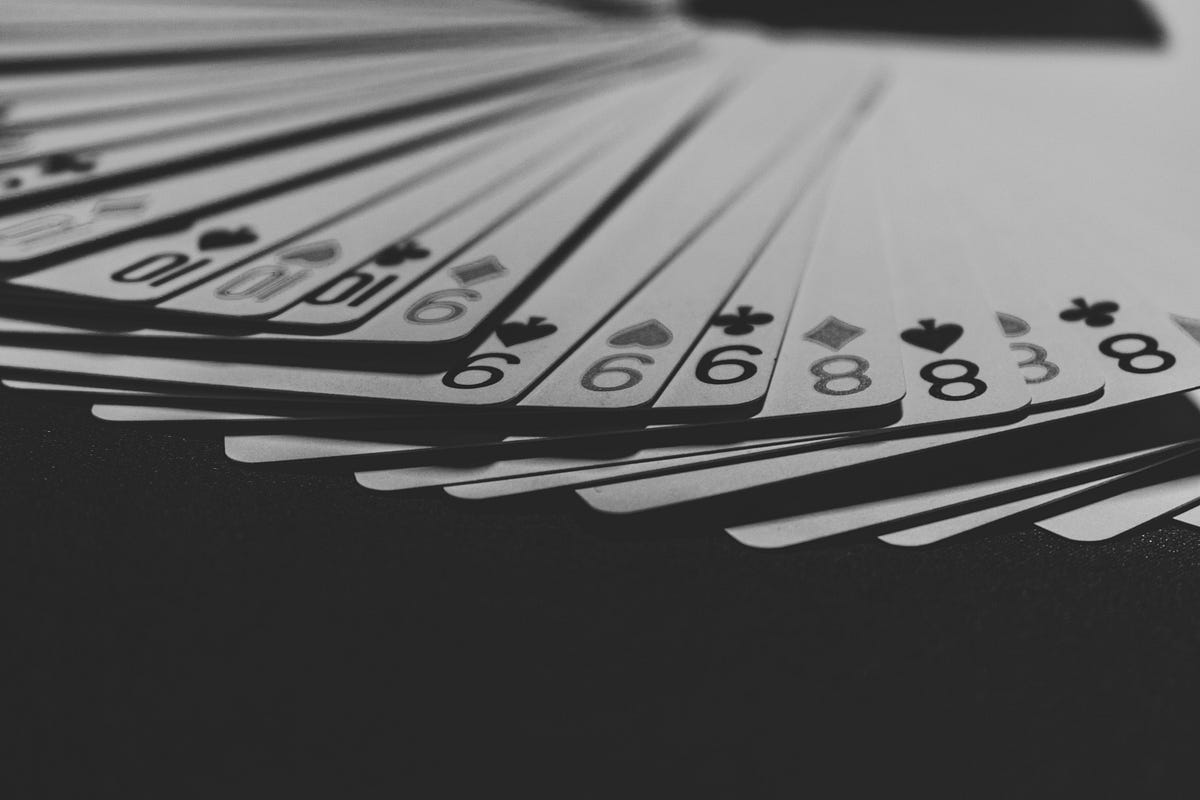1595096220

## Factors That Can Contribute to the Faulty Statistical Inference

Hypothesis testing is a procedure where researchers make a precise statement based on their findings or data. Then, they collect evidence to falsify that precise statement or claim. This precise statement or claim is called the null hypothesis. If the evidence is strong to falsify the null hypothesis, we can reject the null hypothesis and adapt the alternative hypothesis. This is the basic idea of hypothesis testing.

## Error Types in Statistical Testing

There are two distinct types of errors that can occur in formal hypothesis testing. They are:

Type I: Type I error occurs when the null hypothesis is true but the hypothesis testing results show the evidence to reject it. This is called a false positive.

Type II: Type II error occurs when the null hypothesis is not true but it is not rejected in hypothesis testing.

Most hypothesis testing procedure performs well controlling type I error (at 5%) in ideal conditions. That may give a false idea that there is only a 5% probability that the reported findings are wrong. But it’s not that simple. The probability can be much higher than 5%.

## Normality of the Data

The normality of the data is an issue that can break down a statistical test. If the dataset is small, the normality of the data is very important for some statistical processes such as confidence interval or p-test. But if the data is large enough, normality does not have a significant impact.

## Correlation

If the variables in the dataset are correlated with each other, that may result in poor statistical inference. Look at this picture below: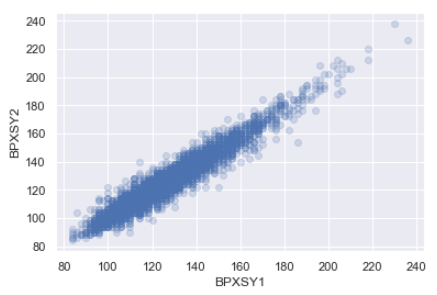In this graph, two variables seem to have a strong correlation. Or, if a series of data is observed as a sequence, that means values are correlated with its neighbors, and there may have some clustering or autocorrelation in the data. This kind of behavior in the dataset can adversely impact the statistical tests.

## Correlation and Causation

This is especially important when interpreting the result of a statistical test. “Correlation does not mean causation”. Here is an example. Suppose, you have study data that shows, more people who do not have college education believe that women should get paid less than men in the workplace. You may have conducted a good hypothesis testing and prove that. But care must be taken on what conclusion is drawn from this. Probably, there is a correlation between college education and the belief that ‘women should get paid less’. But it is not fair to say that not having a college degree is the cause of such belief. This is a correlation but not a direct cause ad effect relationship.

A more clear example can be provided from medical data. Studies showed that people with fewer cavities are less likely to get heart disease. You may have enough data to statistically prove that but you actually cannot say that the dental cavity causes heart disease. There is no medical theory like that.

#statistical-analysis #statistics #statistical-inference #math #data analysis1596675060

## The statistics of Blackjack

Considered as the casino game with the lowest edge for the house, gamblers are supposed to have more chances at winning at Blackjack than at any other game. But what are the odds? And how do we find the optimal strategy to apply?

Let’s find out through a simple statistical analysis!

# Setting the scene

This article exploits a simulation tool for Blackjack built with **R **to deduce an optimal strategy and the associated probabilities.

All codes are available on Github.

## ArnaudBu/blackjack_simulation

Simulations of blackjack games. Contribute to ArnaudBu/blackjack_simulation development by creating an account on…

github.com

The rules used for the simulations are:

• Each player, including the dealer, starts with two cards. One of the dealer’s cards is hidden and will be revealed at the end of the round, when comes the dealer’s turn to play.
• The goal is to ask for cards to beat the dealer’s hand without exceeding 21, each card accounting for its nominal value (Kings, Queens, and Jacks are worth 10). Aces are worth 1 or 11, whichever value gives the best score without busting. If a hand has an ace whose value is 11, it is called soft, and the opposite is called a hard hand.
• If the player exceeds 21 (bust), no matter the dealer’s score, the dealer wins the bet. If the dealer busts and the player does not, the player wins. In case of similar scores under 21, the round results in a draw. In all other cases, the higher score wins the round.
• The dealer pays the bet 1 to 1, except for a natural Blackjack (Ace + card whose value is 10) that pays 3 to 2.
• At each move, the player can _hit _(ask for a card), _stand _(remain at its current position), or _double _(the bet is doubled but only one more card will be drawn).
• When the player has two identical cards, he can split, which means he can transform his pair into two separate hands that will be played independently.

The script `[run.R](https://github.com/ArnaudBu/blackjack_simulation/blob/master/run.R)` simulates Blackjack games (based on 10,000,000 moves with 8 decks and 3 people playing) to generate a database that will be analyzed with the data.table package.

The dataset produced follows the format below, with each line representing a move in a round (game_id) with the expected earning in case the player hits, stands, or doubles. The hard_if_hit value indicates if the hand is hard after the move and is necessary for the optimal strategy definition.

``````	score	score_dealer	hard	score_if_hit	score_fin_dealer	game_id	hit	stand	double	hard_if_hit
5	10	TRUE	11	19	4	-1	-1	-2	TRUE
11	10	TRUE	20	19	4	1	-1	2	TRUE
20	10	TRUE	30	19	4	-1	1	-2	TRUE
14	10	TRUE	24	19	5	-1	-1	-2	TRUE
14	9	TRUE	24	19	6	-1	-1	-2	TRUE
15	9	FALSE	19	19	7	0	-1	0	FALSE
19	9	FALSE	16	19	7	-1	0	-2	TRUE
16	9	TRUE	18	19	7	-1	-1	-2	TRUE
18	9	TRUE	19	19	7	0	-1	0	TRUE
19	9	TRUE	29	19	7	-1	0	-2	TRUE
view raw
blackjack_games_examples.csv hosted with ❤ by GitHub
``````

Table 1 — Blackjack analytics dataset

All analyses performed are available in the `[analysis.R](https://github.com/ArnaudBu/blackjack_simulation/blob/master/analysis.R)` script.

# Basic strategy

The most basic strategy one could think about is standing once the score reaches a certain threshold.

In terms of code, we just need to filter the dataset to retrieve one line by _game_id _that corresponds to the first score that exceeds the threshold, or the first _score_if_hit _that does so if the first condition is not met.

Applying this strategy to the dataset for each threshold from 2 to 21, we get the following results after aggregation.Figure 1 — Hit threshold strategy returns

The graph above represents the expected earnings for each round (in percent of the gambled amount) with the distribution of outcomes.

The optimal strategy uses a threshold of 15, for an expected loss of 8.57 % of the gambled amount at each round. Those odds would obviously lead the game to end prematurely for most players.

It is, fortunately, possible to design a strategy capable of improving these odds by taking into account the dealer’s score and the soft aces in the player’s hand in the decision process.

# The optimal strategy

To find the optimal strategy, we first need a metric for optimization. We will use the expected earnings after a move.

Computing this metric for a given hand (score & if the hand is soft), requires knowing:

• the next possible hands when hitting, with the probability to transition to each one of them;
• the associated expected earnings for those hands, based on the moves given by the strategy we aim at defining.

We thus have a recursive problem to handle, since we first need to estimate the possible later moves of the round, dealer’s score being constant. This requires implementing a loop going** backward on scores. **Nevertheless, the possibility to have a hard or soft hand implies to also consider this when ordering the steps.

The following chart describes the transitions between hands.Figure 2 — Possibilities when hitting

If the player has a hard hand with a score higher than 10, he can only get a hard hand with a higher score when hitting. A soft hand can transform into a soft hand with a higher score or a hard hand with a score higher than 10. Finally, a hard hand with a score lower than 9 can give a soft hand or a hard hand with a higher score. This means that we need to sequence our backward loop with three pivotal steps.

#data-analytics #r #simulation #blackjack #data-visualization #data analysis1594278000

## Confidence Interval, Calculation, and Characteristics

The Confidence Interval (CI) is very important in statistics and data science. In this article, I am going to explain the confidence interval, how to calculate it, and the important characteristics of it.

The confidence interval (CI) is a range of values. It is expressed as a percentage and is expected to contain the best estimate of a statistical parameter. A confidence interval of 95% mean, it is 95% certain that our population parameter lies in between this confidence interval.

## Interpretation of confidence intervals

Here is a statement:

“In a sample of 659 parents with toddlers, 540, about 85 percent, stated they use a car seat for all travel with their toddler. From these results, a 95% confidence interval was provided, going from about 82.3 percent up to 87.7 percent.”

This statement means, it is 95% certain that the population proportion that uses a car seat for all travel with their toddler is 82.3 and 87.7. If we take several subsamples from this population, 95% of the time, the population proportion that uses a car seat for all travel with their toddler will fall between 82.3% to 87.7%.

Can we say that the confidence interval (82.3, 87.7) contains the true population proportion? The answer is unknown. The population proportion is a fixed value but unknown. It is important to remember that 95% confidence does not mean a 95% probability.

## Why Confidence Interval Is Important?

It is important because it is not possible to take data from every single person in a population most of the time. In the example above, the sample size was 659. We estimated the population proportion of the parents with toddlers who use a car seat for all travel from a sample of 659 parents. We could not get the data from all the parents with toddlers. So, we calculate the population proportion from our available sample and consider a margin of error. With that margin of error, we get a range. This range is called a confidence interval. A confidence interval is a way to express how well the sample data represent the total population. You can calculate a confidence interval of any number(less than 100%). But a 95% confidence interval is the most common.

## How to Calculate the Confidence Interval

The formula for the confidence interval is: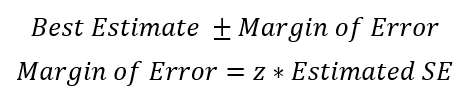We normally want a high confidence level such as 75%, 95%, or 99%. Higher the confidence level(CL), lower the precision. In the example above, the best estimate is 85%. We can calculate the estimates SE from the following formula: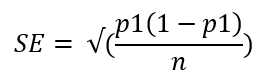In the equation, above p1 is the best estimate and n is the sample size. Here is a table for z- score for a few commonly used confidence level.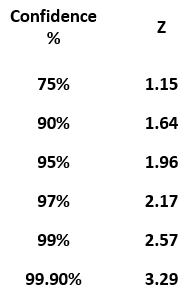Plugging in all the values,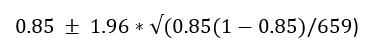The confidence interval come out to be 82.3% and 87.7%.

## The range of CI is higher for higher CL

In the same way, we can calculate a 99% confidence level. You only need to change the z-score. From the table above, the z-score for a 99% confidence level is 2.57. Plugging in that value in the confidence interval formula, the confidence interval for a 99% confidence level is 81.43% to 88.57%. The range of a confidence interval is higher for a higher confidence level.

#statistical-analysis #confidence-interval #statistics #statistical-learning #data-science #data analysis1603098000

## Introduction

In this article, I will show how missing values can lead to biased estimates by working through a common dataset in 4 scenarios where the missing value mechanism differs. The content in this article is based on Chapter 15 in .The code to reproduce the results in this article can be found in this notebook.I assume the reader is familiar with building generalized linear models (GLMs) and using directed acyclic graphs (DAGs) to illustrate causality.

## Dataset

Suppose we want to study the effect of student diligence and homework quality. Let’s imagine that we were somehow able to assign a real number to measure a student’s diligence and that homework quality is measured on a 10-point scale, 0 to 10.In other words, our dataset looks like this: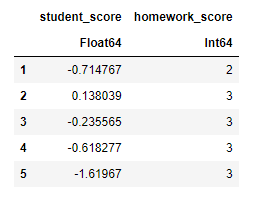Figure 1: Sample synthetic dataset

A student’s student score is just a random variable sampled from a standard normal distribution.

#statistical-inference #data-science #mathematics #statistics #bayesian-statistics1603148400

## How is Sample Size Related to Standard Error, Power, Confidence Level, and Effect Size?

When conducting statistical analysis, especially during experimental design, one practical issue that one cannot avoid is to determine the sample size for the experiment. For example, when designing the layout of a web page, we want to know whether increasing the size of the click button will increase the click-through probability. In this case, AB testing is an experimental method that is commonly used to solve this problem.

Moving to the details of this experiment, you will first decide how many users I will need to assign to the experiment group, and how many we need for the control group. The sample size is closely related to four variables, standard error of the sample, statistical power, confidence level, and the effect size of this experiment.

In this article, we will demonstrate their relationships with the sample size by graphs. Specifically, we will discuss different scenarios with one-tail hypothesis testing.

Standard Error and Sample Size

The standard error of a statistic corresponds with the standard deviation of a parameter. Since it is nearly impossible to know the population distribution in most cases, we can estimate the standard deviation of a parameter by calculating the standard error of a sampling distribution. The standard error measures the dispersion of the distribution. As the sample size gets larger, the dispersion gets smaller, and the mean of the distribution is closer to the population mean (Central Limit Theory). Thus, the sample size is negatively correlated with the standard error of a sample. The graph below shows how distributions shape differently with different sample sizes

#statistics #statistical-power #statistical-analysis #data-science #sample-size